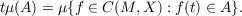#### Vol. 69, No. 1, 1977

 Download this articleFor screen For printingRecent Issues Vol. 325: 1  2 Vol. 324: 1  2 Vol. 323: 1  2 Vol. 322: 1  2 Vol. 321: 1  2 Vol. 320: 1  2 Vol. 319: 1  2 Vol. 318: 1  2Online Archive Volume: Issue:The Journal Subscriptions Editorial Board Officers Contacts Submission Guidelines Submission Form Policies for Authors ISSN: 1945-5844 (e-only) ISSN: 0030-8730 (print) Special Issues Author Index To Appear Other MSP Journals
Measures with continuous image law

### Sun Man Chang

Vol. 69 (1977), No. 1, 25–36
##### Abstract

Let M be a topological space, and X a metric space. Let P(X) denote the collection of probability measures on X. Let C(M,X) denote the set of continuous functions from M to X. Let P(X) have the weak topology, and let C(M,X) have the topology of uniform convergence. For a fixed measure μ P(C(M,X)), and a member t M, define a measure on X byIn this paper, we consider the following problem: given a continuous function T : M P(X), when is there a measure μ P(C(M,X)) such that T(t) = for all t?

##### Mathematical Subject Classification 2000
Primary: 28A32, 28A32
Secondary: 46E30
##### Milestones